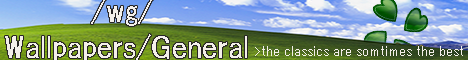[a / b / c / d / e / f / g / gif / h / hr / k / m / o / p / r / s / t / u / v / vg / vr / w / wg] [i / ic] [r9k / s4s / vip / qa] [cm / hm / lgbt / y] [3 / aco / adv / an / asp / bant / biz / cgl / ck / co / diy / fa / fit / gd / hc / his / int / jp / lit / mlp / mu / n / news / out / po / pol / qst / sci / soc / sp / tg / toy / trv / tv / vp / wsg / wsr / x] [Settings] [Search] [Home]
Board
/sci/ - Science & MathWhat is a "homomorphism of rings?" A homomorphism is just some sort of map that preserves the structure of two groups, right? I don't even know where to begin this shit, FUCK.
>>
In this context, it's clearly not a group homomorphism, it's a ring homomorphism. They even just straight up define it. All you have to show is that this inclusion map (identity with domain restricted to a subring) is a ring homomorphism.
To show it's not a isomorphism for proper subrings, it should be obvious that a proper subset and the full set can't be isomorphic.
>>
>>10800025
God I wish I could understand what the fuck you were even saying. They straight up define what?
>>
consider the function f(x) =x for all x in S.
let x,y be in S then
f(x + y ) = x + y = f(x) + f(y)
f(x*y) = x*y = f(x)*f(y)
f(1_S) = 1_S = 1_R since S is a subring
Therefore f is a ring homomorphism S->R since x,y are also in R
>>
>>10800046
That's what I did but it seemed too simple...
>>
>>10800053
yeah, algebra is easy mode once you understand the nomenclature
>>
>>10800013
A ring homomorphism is just a function f(x) that takes elements of one ring and gives out an element of another ring, while also satisfying:
>f(x + y) = f(x) + f(y)
>f(x * y) = f(x) * f(y)
>f(1) = 1 (where 1 is just the multiplicative identity)

For (a), another anon gave a good explanation - it's incredibly simple, but it's designed to test if you actually understand what you're supposed to be doing. It's like asking what 1+2 is. It's a simple question, but doing it shows that you understand that it's addition and not multiplication.

A ring isomorphism g(x) is a ring homomorphism that is ALSO bijective, i.e.:
>injective or one-to-one: g(x) = g(y) ==> x=y
>surjective or onto: for any element r of the domain R (the second ring that the function gives out values for), you have some element x in S such that g(x) = r. Interestingly, this means that whether or not a function is surjective is only determined by how you define the domain of the function. For instance, sin : R -> [-1, 1] is surjective, but sin : R -> R is not.

Thus, to show that phi (I'll just use p(x)) is not an isomorphism, you need it to simply not satisfy ONE of those conditions. Specifically for (b), we will be using a proof by contradiction:
>suppose that p(x) : S -> R with p(x) = x for all s in S is an isomorphism
>since S is a proper subring of R, there exists some element u in R such that u is not in S
>since u is in R and p is surjective, there exists some element s in S such that p(s) = u
>however, since p(s) = s for any s in S, we must have s=u, which contradicts our statement that u is not in S
>thus, p cannot be an isomorphism
>>
>>10800013
Is this peak retardation?
>>10800053
Jesus Christ it is.
>>
>>10801207
i literally solved it you retard
next

Delete Post: [File Only] Style: